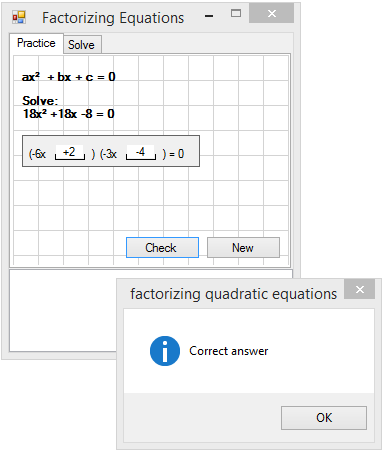#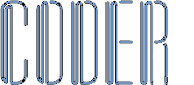Maths Revision V2.0

This is my second suite of 22 revision aid programs + tools I wrote...

(Click the icons to navigate)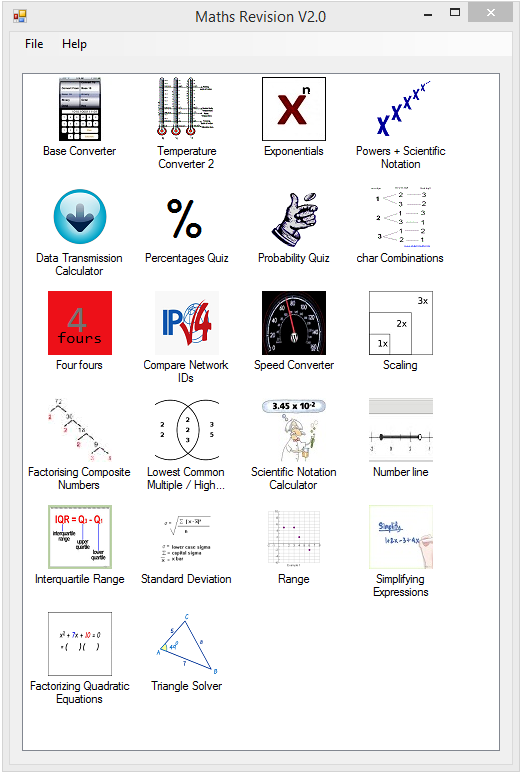This is a numeric base converter that converts numbers from one base to another: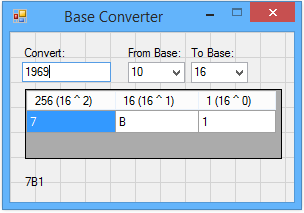This is an update to my last version of my Temperature Converter, that converts to and from Celsius, Fahrenheit, and Kelvin: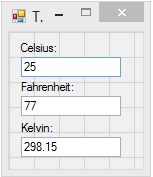This is a simple program that demonstrates raising 10 to an integer power: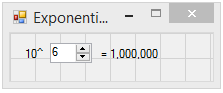This is my Powers and Scientific Notation quiz program: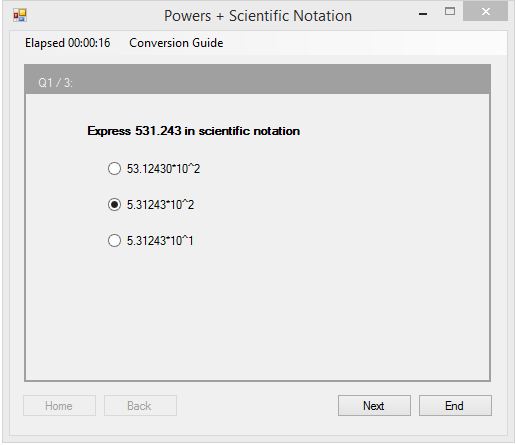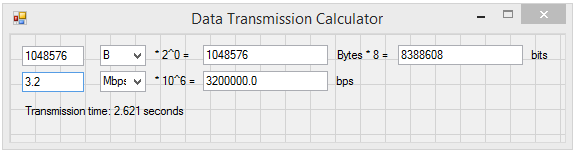This is a Percentages quiz, where you must calculate the percentage of one number that results in another number: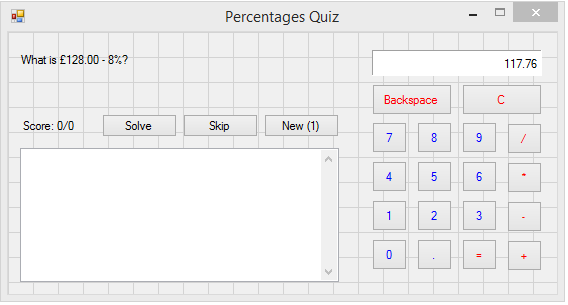This is a Probability quiz, where you must calculate the odds of drawing a specified number of colored balls from a bag: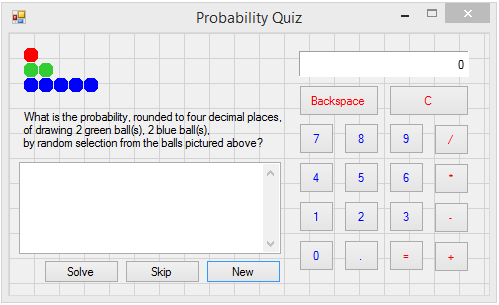This is a char combinations program which calculates and shows all possible combinations of a user entered set of characters: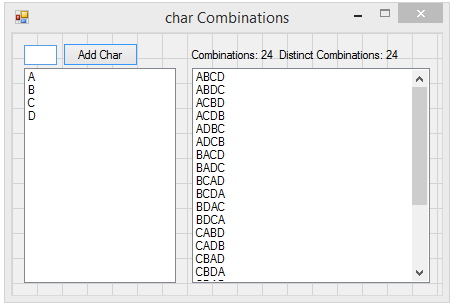This is a Four fours game where you must use four of the number 4 with any of the arithmetic operators (+,-,/,*) and optionally brackets () and perform calculations that result in the numbers 1 to 10. Four 4s must be used to calculate each number: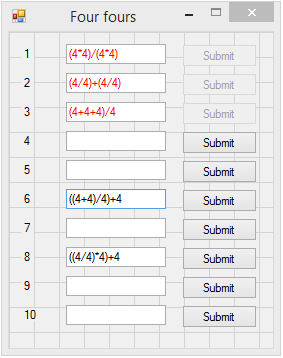My Compare Network IDs program compares two IP Addresses to determine if they have the same Network ID: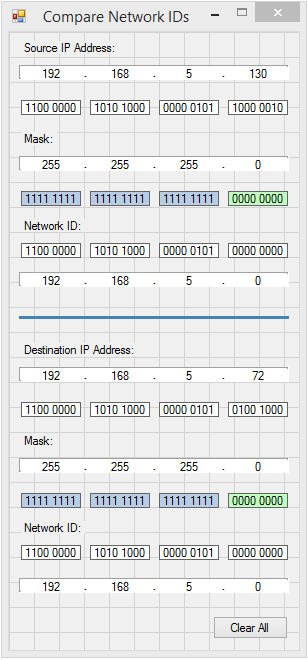This program is a Speed Converter that converts between m/s and km/h and vice versa:This is a Scaling program for map distance conversions: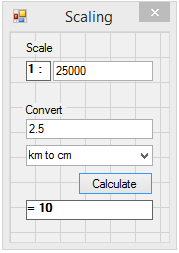This is a Factorising Composite Numbers program that shows a Factor tree: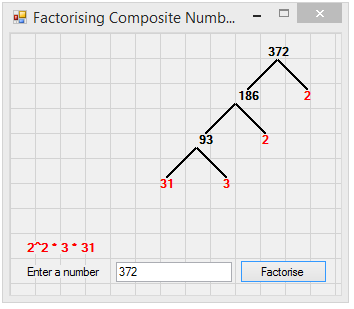This is a Lowest Common Multiple / Highest Common Factor program that factorizes two numbers showing a graphical output:This is a Scientific Notation Calculator that performs arithmetrical operations on two Scientific Notation numbers: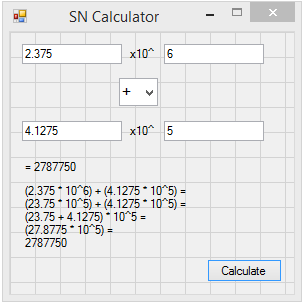This is a Number Line creator that draws and saves a dynamic Number Line: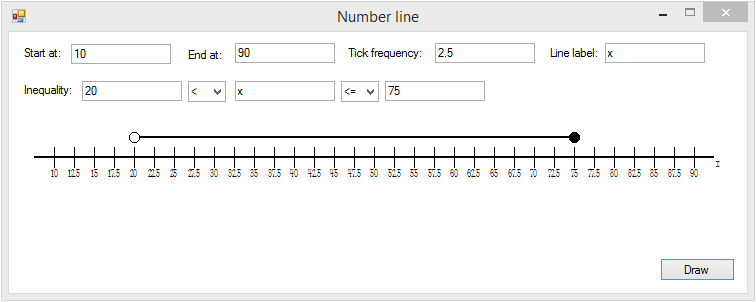This is a simple Interquartile Range calculator:This is a simple Standard Deviation calculator: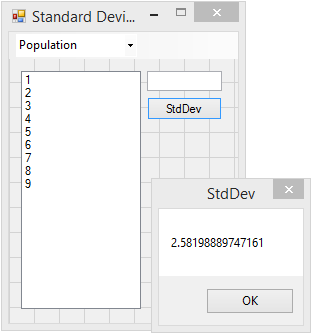This is a simple Range calculator: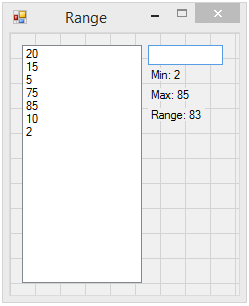This is a Simplifying Expressions program: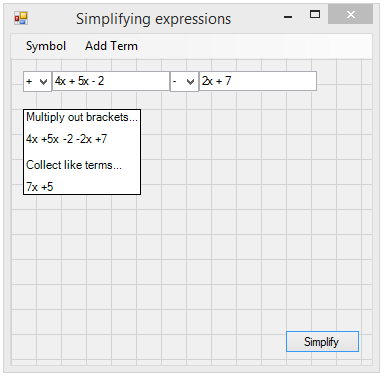This is a Factorizing Quadratic Equations solver: